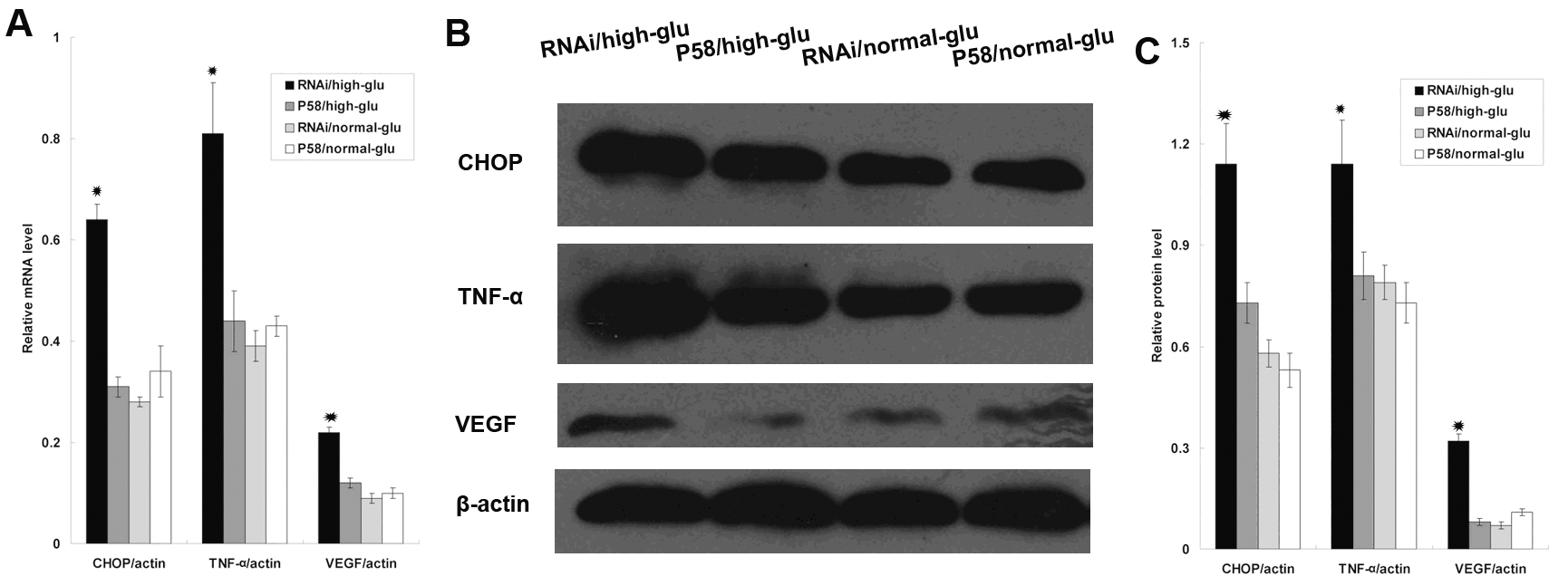Figure 4 of Yang, Mol Vis 2011; 17:78-84.

Figure 4. Overexpression of P58IPK suppressed the expression of CHOP, TNF-α, and VEGF in cultured RRCECs. A: mRNA levels of CHOP, TNF-α, and VEGF were determined by real-time PCR, in GFP/dia group, GFP/no-dia group, P58IPK/dia group, and P58IPK/no-dia group. The expression level of CHOP in each groups were 0.64 ±0.03, 0.31±0.02, 0.28±0.01 and 0.34±0.05;respectively, (p<0.05, n=6), The expression level of TNF-α in each groups were 0.81±0.10, 0.44± 0.06, 0.39±0.03, and 0.43±0.02 respectively, (p<0.05, n=6), The expression level of VEGF in each groups were 0.22±0.01, 0.12±0.01, 0.09±0.01, and 0.10±0.01 respectively, (p<0.05, n=6), B: A representative western blot result is shown in B. C: The amount of protein expression was quantified relative to the level of β-actin. Results are representative of six experiments. Results are expressed as mean±standard deviation; The protein level of CHOP in each groups were 1.14 ±0.12, 0.73±0.06, 0.58±0.04, and 0.53±0.05; respectively, (p<0.05, n=6), The protein level of TNF-α in each groups were 1.41±0.13, 0.81±0.07, 0.79±0.05, and 0.73±0.06 respectively, (p<0.05, n=6), The protein level of VEGF in each groups were 0.32±0.02, 0.08±0.01, 0.07±0.01 and 0.11±0.01 respectively, (p<0.05, n=6).